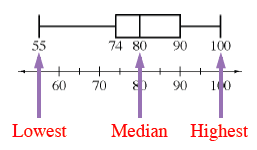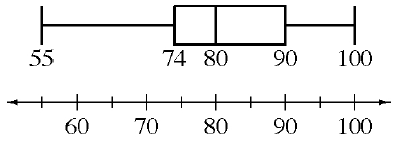### Home > CC3 > Chapter Ch6 > Lesson 6.2.2 > Problem6-63

6-63.

The box plot at right shows the different grades (in percents) that students in Ms. Sanchez’s class earned on a recent test.

1. What was the median score on the test? What were the highest and lowest scores?

Do you remember how to read a box plot?1. Did most students earn a particular score? How do you know?

Can this be determined from a box plot?

1. If Ms. Sanchez has $32$ students in her class, about how many students earned a grade of $80\%$ or higher? About how many earned more than $90\%$? Explain how you know.

Since $80\%$ is the median, half of the class earned $80\%$ or higher and half earned lower than $80\%$.

$\frac{1}{4}\text{ of the class earned 90%}$

$16$ students earned $80\%$ or higher, $8$ students earned $90\%$ or higher

1. Can you tell if the scores between $80\%$ and $90\%$ were closer to $80\%$ or closer to 90%? Explain.

Can this be determined from a box plot?